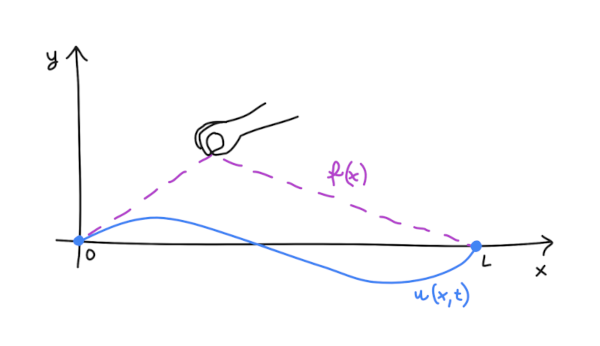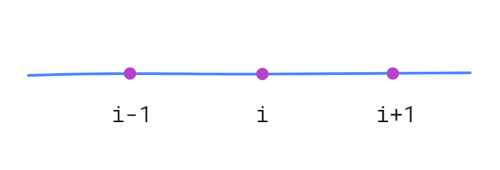### Vibrating string equation (without damping)Vibrating string without damping is represented by the following differential equation:

$$\begin{cases} \begin{array}{l@{\ }l@{\ }l} \frac{{{\partial ^2}u}}{{\partial {t^2}}} = {a^2}\frac{{{\partial ^2}u}}{{\partial {x^2}}} , & \hspace{0.25in} 0 \leqslant x \leqslant L , 0 \leqslant t \leqslant \infty , & \hspace{0.25in} \text{string equation} ; \\ u\left( {0,t} \right) = u\left( {L,t} \right) = 0 , & \hspace{0.25in} 0 \leqslant t \leqslant \infty , & \hspace{0.25in} \text{boundary conditions} ; \\ u\left( {x,0} \right) = f\left( x \right) , \frac{{\partial u}}{{\partial t}}\left( {x,0} \right) = 0 , & \hspace{0.25in} 0 \leqslant x \leqslant L , & \hspace{0.25in} \text{initial conditions}; \end{array} \end{cases} \tag{1}$$### Splitting the string equation into two coupled equations

We need to transform the equation:

$$\frac{{{\partial ^2}u}}{{\partial {t^2}}} = {a^2}\frac{{{\partial ^2}u}}{{\partial {x^2}}} \tag{2}$$

Let's introduce E value, which represents time derivative of the y-position:

$$E = \frac{{{\partial}u}}{{\partial {t}}} \tag{3}$$

So now, we've got two coupled equations:

$$\begin{cases} \begin{array}{l@{\ }l@{\ }l} \frac{{{\partial}E}}{{\partial {t}}} = {a^2}\frac{{{\partial ^2}u}}{{\partial {x^2}}} \\ \frac{{{\partial}u}}{{\partial {t}}} = E \end{array} \end{cases} \tag{4}$$

We will use them in the numerical solution.

### Eulerian mesh

Current state of the vibrating string we will store in an 1-dimentional differential Eulerian mesh. We will store there finite number of points of the string and for each point its current y-position (u) and its time derivative of the y-position (E), which is in fact the current velocity of the particular point of the string.To implement it, we just need to create two 1-dimensional arrays: U and E. Lenght of both arrays is the same and represents number of points of the string. Number of points is up to us. More points - more accurate calculations, but cost of calculations bigger.

### Numerical solution

In numerical solution we will calculate state of the string (u and E) for the next time-step (time n+1) basing on current state of the string (time n).

Numerical solution for the cupled equations (4) looks like this:

$$\begin{cases} \begin{array}{l@{\ }l@{\ }l} E^{n+1}_{i} = E^{n}_{i} + {a^2} \frac{ u^{n}_{i+1} - 2 u^{n}_{i} + u^{n}_{i-1} }{ \Delta x^{2} } \Delta t \\ u^{n+1}_{i} = u^{n}_{i} + E^{n+1}_{i} \Delta t \end{array} \end{cases} \tag{5}$$

In the first equation there was used the second-order central difference approximation. In the second - linear dependence.

In code of my simulation this fragment looks like this:


_calculateNextStep () {
let currentE = this.eulerianMesh['e'];
let currentU = this.eulerianMesh['u'];
let nextE = [];
let nextU = [];

// Apply boundary conditions
nextE = 0;
nextE[this.pointsNumb - 1] = 0;
nextU = 0;
nextU[this.pointsNumb - 1] = 0;

// Calculations for other points
for (let i = 1; i < (this.pointsNumb - 1); i++) {
nextE[i] = currentE[i] + Math.pow(this.v, 2) * ((currentU[i+1] - 2*currentU[i] + currentU[i-1]) / Math.pow(this.deltaX, 2)) * this.deltaT;
}
for (let i = 1; i < (this.pointsNumb - 1); i++) {
nextU[i] = currentU[i] + nextE[i] * this.deltaT;
}

this.eulerianMesh = {
'e': nextE,
'u': nextU,
};
}


### Initial conditions

As an initial state of the Eulerian mesh we have to set:

• u: y-positions of the points for the initial shape of the string
• E: fill with zeros, because velocity of each point of the string equals zero before we release tensioned string

### Simulation

You can play with the simulation here or by clicking the image below:

You can compare bahavior of the string from the simulation with the real experiment: YouTube: Motion of Plucked String

### GitHub

Code of the simulation is available on GitHub here https://github.com/tomdwor/vibrating-string

### References

• Markowe Wykłady z Matematyki: równania różniczkowe, Marek Zakrzewski, GiS 2020
• Symulacje komputerowe w fizyce, Maciej Matyka, Helion 2021